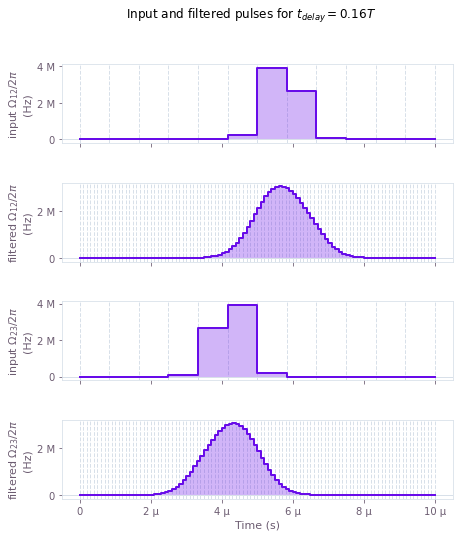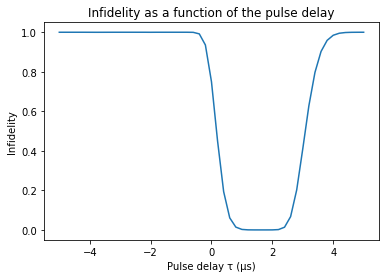# Simulation

Simulating the dynamics of quantum systems in the presence of noise

The Q-CTRL Python package enables you to simulate the dynamics of quantum systems that are affected by various noise processes. Such simulations can provide useful insights into the expected real-world performance of candidate control solutions. In this notebook we show how to use the Q-CTRL Python package to perform simulations of systems affected by different types of noise.

## Imports and initialization

All usage of the Q-CTRL Python package begins by importing the qctrl package and starting a session.

# Essential imports
import numpy as np
from qctrl import Qctrl

# Predefined pulse imports
from qctrlopencontrols import new_predefined_driven_control

# Plotting imports
import matplotlib.pyplot as plt
from qctrlvisualizer import plot_controls

# Starting a session with the API
qctrl = Qctrl()


## Worked example: single qubit subject to dephasing noise

In this example we will show how to simulate the dynamics of a single qubit experiencing stochastic dephasing noise, driven by a $\pi/2$ CORPSE pulse. The Hamiltonian of the quantum system is:

\begin{align*} H(t) = & \frac{\Omega(t)}{2} \sigma_- + \frac{\Omega^*(t)}{2} \sigma_+ + \frac{\eta(t)}{2} \sigma_z, \end{align*}

where $\Omega(t)$ is a time-dependent Rabi rate, $\eta(t)$ is a stochastic dephasing noise process, $\sigma_\pm = (\sigma_x \mp i \sigma_y)/2$, and $\sigma_k$ are the Pauli matrices.

We assume that $\eta(t)$ represents pink noise, as defined below.

def pink_spectrum(frequencies, frequency_cutoff):
return 1/(frequencies + frequency_cutoff)

frequencies = np.linspace(0, 2*1e6, 1000)

power_densities = 4e9*pink_spectrum(frequencies=frequencies, frequency_cutoff=0.05*1e6)

plt.plot(frequencies/1e6, power_densities*1e6, c="#FB00A5")
plt.fill_between(frequencies/1e6, 0, power_densities*1e6, alpha=0.25, color="#FB00A5")
plt.xlabel("Frequency (MHz)")
plt.ylabel("Power density (1/MHz)")
plt.title("Dephasing noise spectral density")
plt.show()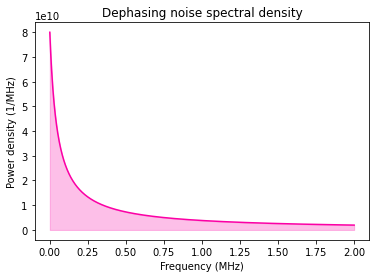We now proceed to show how the system, driven by stochastic noise drawn from this power spectral density, may be simulated using the Q-CTRL Python package.

### Creating the pulses

As described in the Setup user guide, we first set up Python objects representing the pulse, control, and noise.

The qctrl.functions.calculate_colored_noise_simulation function accepts the Hamiltonian in the form of separate terms for each of the complex-valued controls (drives), real-valued controls (shifts), and static terms (drifts). In the Hamiltonian used in this example, the drive corresponds to the term that contains the Rabi rate ($\Omega(t)$ and $\Omega^*(t)$) and the drift corresponds to the dephasing term (there are no shifts).

The Rabi coupling term is defined using a pulse from Q-CTRL Open Controls. To create the dephasing noise term, we provide its noise spectral density as a qctrl.types.colored_noise_simulation.Noise by using the frequencies and power densities defined above.

# Define standard matrices
identity = np.array([[1, 0],[0, 1]], dtype=np.complex)
sigma_x = np.array([[0, 1],[1, 0]], dtype=np.complex)
sigma_z = np.array([[1, 0],[0, -1]], dtype=np.complex)
sigma_m = np.array([[0, 1],[0, 0]], dtype=np.complex)
square_root_sigma_x = 0.5*np.array([[1+1j, 1-1j], [1-1j, 1+1j]], dtype=np.complex)

# Define control parameters
omega_max = 2*np.pi * 1e6 #Hz
total_rotation = np.pi/2

# Define pulse using pulses from Q-CTRL Open Controls
pulse = new_predefined_driven_control(
rabi_rotation=total_rotation,
azimuthal_angle=0.,
maximum_rabi_rate=omega_max,
scheme='CORPSE',
name='CORPSE',
)

# Define Rabi coupling term
rabi_drive = qctrl.types.colored_noise_simulation.Drive(
control=[
qctrl.types.ComplexSegmentInput(duration=d, value=v)
for d, v in zip(pulse.durations, pulse.rabi_rates * np.exp(1j*pulse.azimuthal_angles))],
operator=sigma_m/2,
)

# Define dephasing noise term
noise_drift = qctrl.types.colored_noise_simulation.Drift(
operator=sigma_z,
noise=qctrl.types.colored_noise_simulation.Noise(
power_densities=power_densities,
frequency_step=frequencies,
time_domain_sample_count=100,
),
)


### Running the simulation

The computation of a simulation with colored noise is carried out by the qctrl.functions.calculate_colored_noise_simulation function, which takes the following parameters:

• duration, the duration of the control pulses,
• sample_times, which is a list of sample times at which the dynamics should be calculated,
• drives, shifts, and drifts, which represent the different terms in the Hamiltonian (at least one term needs to be provided),
• trajectory_count, which is the number of independent simulations to run using different random realizations of the noise processes,
• initial_state_vector, which gives an initial state vector to be propagated according to the calculated dynamics (optional), and
• target, which gives the target time evolution operator with respect to which the operational infidelities will be calculated throughout the simulated time period (optional).
# Define sample times for the output
sample_times = np.linspace(0, pulse.duration, 100)

# Define the target (optional)
target = qctrl.types.TargetInput(operator=square_root_sigma_x)

colored_noise_simulation_result = qctrl.functions.calculate_colored_noise_simulation(
duration=pulse.duration,
sample_times=sample_times,
drives=[rabi_drive],
drifts=[noise_drift],
trajectory_count=5,
initial_state_vector=np.array([1., 0.]),
target=target,
)

100%|██████████| 100/100 [00:04<00:00, 23.44it/s]


### Extracting the average infidelities

After the calculation of all the noisy trajectories, a colored noise simulation returns different types of data. Here we show how to extract the average infidelity, which gives the operational infidelity of the time evolution operator relative to the specified target, averaged over the ensemble of trajectories. All average quantities are stored in colored_noise_simulation_result.average_samples for each time in the provided sample_times (where colored_noise_simulation_result is the object returned by qctrl.functions.calculate_colored_noise_simulation).

# Extract infidelities
average_infidelities = [
average_sample.average_infidelity
for average_sample in colored_noise_simulation_result.average_samples
]

# Plot infidelities
plt.plot(sample_times*1e6, average_infidelities)
plt.xlabel("Time (µs)")
plt.ylabel("Infidelity")
plt.title("Average infidelity")
plt.show()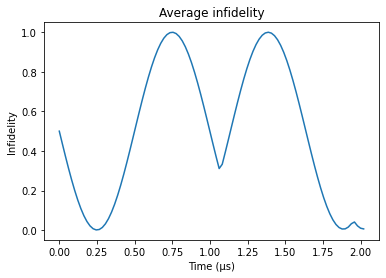### Extracting the average density matrices

colored_noise_simulation_result.average_samples also includes the density matrix, the object describing an ensemble of state vectors for the different trajectories. We can use it (for example) to visualize trajectories of observables.

# Extract density matrices
density_matrices = [
average_sample.average_density_matrix
for average_sample in colored_noise_simulation_result.average_samples
]

# Calculate and plot average sigma_x expectation
plt.plot(
sample_times*1e6,
[
np.real(np.trace(sigma_x.dot(density_matrix)))
for density_matrix in density_matrices
],
)
plt.axhline(y=0., c='k', lw=0.5)
plt.xlabel("Time (µs)")
plt.ylabel(r"$\langle\sigma_x\rangle$")
plt.title(r"Average $\sigma_x$ expectation")
plt.show()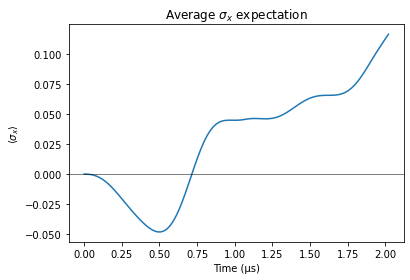### Extracting the individual noise trajectories

In addition to the ensemble information available in the average samples, we can extract information about the individual simulation trajectories. These are stored in colored_noise_simulation_result.trajectories. Here we show how to extract the random realizations of the dephasing noise process.

# Extract noise trajectories
noise_points_list = [
np.array([
segment.value
for segment in trajectory.noise_realizations.segments
])
for trajectory in colored_noise_simulation_result.trajectories
]

# Extract noise sampling times
noise_times = np.cumsum([
segment.duration
for segment in colored_noise_simulation_result.trajectories.noise_realizations.segments
])

# Plot all noise trajectories
for noise_points in noise_points_list:
plt.plot(noise_times*1e6,noise_points/1e6)

plt.xlabel("Time (µs)")
plt.ylabel("Noise value (MHz)")
plt.title("Noise trajectories")
plt.show()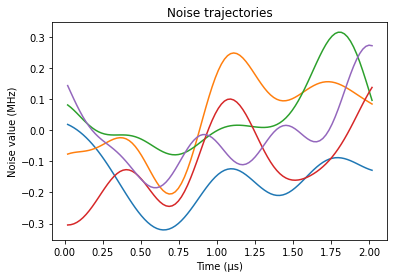### Extracting the individual time evolution trajectories

Next we show how to extract from colored_noise_simulation_result.trajectories the unitary time evolution operators corresponding to each individual simulation run.

# Extract first two time evolution operator trajectories
evolution_operators_list = [
[
sample.evolution_operator
for sample in trajectory.samples
]
for trajectory in colored_noise_simulation_result.trajectories[0:2]
]

# Print initial and final time evolution operators, for both trajectories
print("Time evolution operators at start:")
print(evolution_operators_list)
print(evolution_operators_list)
print("\n")
print("Time evolution operators at end:")
print(evolution_operators_list[-1])
print(evolution_operators_list[-1])

Time evolution operators at start:
[[1.+0.j 0.+0.j]
[0.+0.j 1.+0.j]]
[[1.+0.j 0.+0.j]
[0.+0.j 1.+0.j]]

Time evolution operators at end:
[[ 0.69907233-0.08932679j -0.02472394-0.70901857j]
[ 0.02472394-0.70901857j  0.69907233+0.08932679j]]
[[ 0.70757869-0.05377533j  0.01640906-0.70439432j]
[-0.01640906-0.70439432j  0.70757869+0.05377533j]]


### Summary

We have shown how to use the Q-CTRL Python package to simulate the dynamics of a system in the presence of a stochastic noise process. We have also shown how to extract several quantities of interest from the simulation result, including both ensemble objects describing the average system behavior and objects associated with specific realizations of the stochastic noise process.

## Example: single qubit subject to dephasing noise in a large Hilbert space

In this example we simulate the dynamics of a single qubit experiencing stochastic dephasing noise in the presence of two additional, independent, and unaffected qubits. Since we only care about the average samples here, we can ask to omit the trajectories using the result_scope argument.

The Hamiltonian of the quantum system is:

\begin{align*} H(t) = & \frac{\Omega(t)}{2} \sigma_-^{(1)} + \frac{\Omega^*(t)}{2}\sigma_+^{(1)} + \frac{\eta(t)}{2}\sigma_z^{(1)} \end{align*}

where $\Omega(t)$ is a time-dependent Rabi rate, $\eta(t)$ is a stochastic dephasing noise process, $\sigma_\pm = (\sigma_x \mp i \sigma_y)/2$, and $\sigma_k$ are the Pauli matrices. We use the superscript to indicate that $\sigma^{(1)}$ is the operator for the first qubit and operators for the other two qubits default to the identity matrix.

We assume that $\eta(t)$ represents pink noise, as defined below.

# Define power spectral density
def pink_spectrum(frequencies, frequency_cutoff):
return 1/(frequencies + frequency_cutoff)
frequencies = np.linspace(0, 2*1e6, 1000)
power_densities = 4e9*pink_spectrum(frequencies=frequencies, frequency_cutoff=0.05*1e6)

# Define standard matrices
identity = np.array([[1, 0],[0, 1]], dtype=np.complex)
sigma_x = np.array([[0, 1],[1, 0]], dtype=np.complex)
sigma_z = np.array([[1, 0],[0, -1]], dtype=np.complex)
sigma_m = np.array([[0, 1],[0, 0]], dtype=np.complex)
square_root_sigma_x = 0.5*np.array([[1+1j, 1-1j], [1-1j, 1+1j]], dtype=np.complex)

large_operator = np.array(operator)
large_operator = np.kron(large_operator, identity)
return large_operator

# Define control parameters
omega_max = 2*np.pi * 1e6 #Hz
total_rotation = np.pi/2

# Define pulse using pulses from Q-CTRL Open Controls
pulse = new_predefined_driven_control(
rabi_rotation=total_rotation,
azimuthal_angle=0.,
maximum_rabi_rate=omega_max,
scheme='CORPSE',
name='CORPSE',
)

# Define Rabi coupling term
rabi_drive = qctrl.types.colored_noise_simulation.Drive(
control=[
qctrl.types.ComplexSegmentInput(duration=d, value=v)
for d, v in zip(pulse.durations, pulse.rabi_rates * np.exp(1j*pulse.azimuthal_angles))],
)

# Define dephasing noise term
noise_drift = qctrl.types.colored_noise_simulation.Drift(
noise=qctrl.types.colored_noise_simulation.Noise(
power_densities=power_densities,
frequency_step=frequencies,
time_domain_sample_count=100,
),
)

# Define sample times for the output
sample_times = np.linspace(0, pulse.duration, 100)

# Define the target (optional)

# Run simulation
colored_noise_simulation_result = qctrl.functions.calculate_colored_noise_simulation(
duration=pulse.duration,
sample_times=sample_times,
drives=[rabi_drive],
drifts=[noise_drift],
trajectory_count=50,
initial_state_vector=np.array([1.] + *(len(rabi_drive.operator)-1)),
result_scope=qctrl.types.colored_noise_simulation.ResultScope.NO_TRAJECTORIES,
)

# Extract infidelities
average_infidelities = [
average_sample.average_infidelity
for average_sample in colored_noise_simulation_result.average_samples
]
# Plot infidelities.
plt.plot(sample_times*1e6, average_infidelities)
plt.xlabel("Time (µs)")
plt.ylabel("Infidelity")
plt.title("Average infidelity")
plt.show()  # This should be the same as the infidelity graph above

print("Density matrix at end:")
with np.printoptions(precision=3, linewidth=120, floatmode='maxprec'):
print(colored_noise_simulation_result.average_samples[-1].average_density_matrix)

100%|██████████| 100/100 [00:05<00:00, 17.15it/s]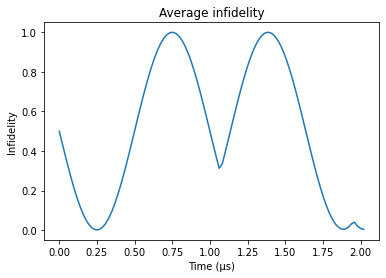Density matrix at end:
[[ 0.499+0.j     0.   +0.j     0.   +0.j     0.   +0.j    -0.005+0.498j  0.   +0.j     0.   +0.j     0.   +0.j   ]
[ 0.   +0.j     0.   +0.j     0.   +0.j     0.   +0.j     0.   +0.j     0.   +0.j     0.   +0.j     0.   +0.j   ]
[ 0.   +0.j     0.   +0.j     0.   +0.j     0.   +0.j     0.   +0.j     0.   +0.j     0.   +0.j     0.   +0.j   ]
[ 0.   +0.j     0.   +0.j     0.   +0.j     0.   +0.j     0.   +0.j     0.   +0.j     0.   +0.j     0.   +0.j   ]
[-0.005-0.498j  0.   +0.j     0.   +0.j     0.   +0.j     0.501+0.j     0.   +0.j     0.   +0.j     0.   +0.j   ]
[ 0.   +0.j     0.   +0.j     0.   +0.j     0.   +0.j     0.   +0.j     0.   +0.j     0.   +0.j     0.   +0.j   ]
[ 0.   +0.j     0.   +0.j     0.   +0.j     0.   +0.j     0.   +0.j     0.   +0.j     0.   +0.j     0.   +0.j   ]
[ 0.   +0.j     0.   +0.j     0.   +0.j     0.   +0.j     0.   +0.j     0.   +0.j     0.   +0.j     0.   +0.j   ]]


## Example: single qubit with leakage

In this example we show how to simulate the dynamics of a single qubit subject to leakage to a third energy level (i.e. a qutrit), driven by a primitive $\pi$ pulse. The qutrit is treated as an oscillator (truncated to three levels) with an anharmonicity of $\chi$, described by the Hamiltonian:

\begin{align*} H(t) = & \frac{\chi}{2} (a^\dagger)^2 a^2 + \frac{\Omega(t)}{2} a + \frac{\Omega^*(t)}{2} a^\dagger, \end{align*}

where $a = \left|0 \right\rangle \left\langle 1 \right| + \sqrt{2} \left|1 \right\rangle \left\langle 2 \right|$ is the lowering operator and $\Omega(t)$ is a time-dependent Rabi rate.

Below we show how to use the Q-CTRL Python package to simulate the time evolution of this coherent system. We also illustrate how the noise-free infidelity $\mathcal{I}_0$ can be extracted from the coherent simulation results. To do this, we choose as target an X gate between the states $\left| 0 \right\rangle$ and $\left| 1 \right\rangle$. Notice that this target is not unitary in the total Hilbert space, but is still a valid target because it is a partial isometry—in other words, it is unitary in the subspace whose basis is $\{ \left| 0 \right\rangle, \left| 1 \right\rangle \}$.

# Define matrices for the Hamiltonian operators
a = np.array([[0., 1., 0.],
[0., 0., np.sqrt(2)],
[0., 0., 0.]], dtype=np.complex)

# Define system parameters
chi = 2*np.pi * 3 * 1e6 #Hz
omega_max = 2*np.pi * 1e6 #Hz
total_rotation = np.pi

# Define pulse using pulses from Q-CTRL Open Controls
pulse = new_predefined_driven_control(
rabi_rotation=total_rotation,
azimuthal_angle=0.,
maximum_rabi_rate=omega_max,
scheme='primitive',
name='primitive',
)

sample_times = np.linspace(0, pulse.duration, 100)

# Define Rabi coupling term
rabi_drive = qctrl.types.coherent_simulation.Drive(
control=[
qctrl.types.ComplexSegmentInput(duration=d, value=v)
for d, v in zip(pulse.durations, pulse.rabi_rates * np.exp(1j*pulse.azimuthal_angles))],
operator=a/2,
)

# Define anharmonic term
anharmonic_drift = qctrl.types.coherent_simulation.Drift(
)

# Define the target
target_operation = np.array([[0, 1, 0],
[1, 0, 0],
[0, 0, 0]], dtype=np.complex)
target = qctrl.types.TargetInput(operator=target_operation)

# Run simulation
coherent_simulation_result = qctrl.functions.calculate_coherent_simulation(
duration=pulse.duration,
sample_times=sample_times,
drives=[rabi_drive],
drifts=[anharmonic_drift],
initial_state_vector=np.array([1., 0., 0.]),
target=target,
)

# Extract and print final infidelity
print("Noise-free infidelity at end: {}".format(coherent_simulation_result.samples[-1].infidelity))

# Extract and print final time evolution operator
print("Time evolution operator at end:")
print(coherent_simulation_result.samples[-1].evolution_operator)

# Extract and plot state populations
times = np.array([
sample.time
for sample in coherent_simulation_result.samples
])
state_vectors = np.array([
sample.state_vector
for sample in coherent_simulation_result.samples
])

for state in range(3):
plt.plot(sample_times*1e6, np.abs(state_vectors[:, state])**2, label=f"P{state}")
plt.xlabel("Time (µs)")
plt.ylabel("Probability")
plt.title("State populations")
plt.legend()
plt.show()

100%|██████████| 100/100 [00:03<00:00, 29.26it/s]
Noise-free infidelity at end: 0.08747804107639123
Time evolution operator at end:
[[ 0.05730981-0.14539786j  0.23505273-0.92588994j -0.08764586+0.23531105j]
[ 0.23505273-0.92588994j -0.06664016+0.18738223j -0.19346042+0.10246022j]
[-0.08764586+0.23531105j -0.19346042+0.10246022j -0.82544823+0.45569409j]]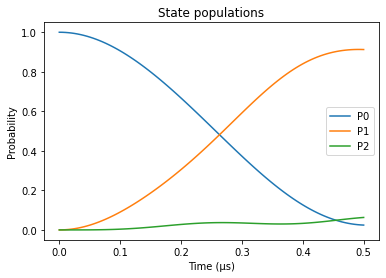## Example: effect of the pulse delay on STIRAP

In this example we show how to simulate the dynamics of a three-level system undergoing stimulated Raman adiabatic passage (STIRAP) when subjected to pump and Stokes pulses. Below we show how to use the Q-CTRL Python package to create and execute a graph made up of nodes (corresponding to the different steps in the simulation) that represent functions of previous nodes (or of constant values). Please see the operations namespace of the Q-CTRL Python package for a list of the currently available primitive nodes.

The Hamiltonian of the system is given by $$H(t) = \frac{1}{2} \Omega_{12}(t) \Big(|1\rangle\langle 2| + |2\rangle\langle 1|\Big) + \frac{1}{2} \Omega_{23}(t) \Big(|2\rangle\langle 3| + |3\rangle\langle 2|\Big) ,$$ where the time-dependent Rabi rates for the pump and Stoke pulses are applied for $t\in(0,T)$ with a Gaussian profile: $$\Omega_{12}(t) = \Omega_\max \exp\left(- \frac{\left(t - \frac{T}{2} - \frac{t_\textrm{delay}}{2}\right)^2}{2\sigma^2}\right) \\ \Omega_{23}(t) = \Omega_\max \exp\left(- \frac{\left(t - \frac{T}{2} + \frac{t_\textrm{delay}}{2}\right)^2}{2\sigma^2}\right)$$ with a maximum amplitude $\Omega_\max$, a pulse width $\sigma$, and a delay between them of $t_\textrm{delay}$.

If the pulses are applied in a counter-intuitive manner, that is, $\Omega_{23}(t)$ is applied before $\Omega_{12}(t)$ (or $t_\textrm{delay} >0$), the STIRAP pulse sequence will transfer the population in state $|1\rangle$ to state $|3\rangle$. In the calculation below, we will see the effect of $t_\textrm{delay}$ on the infidelity of the process. Moreover, we will assume that the input controls are very coarse, consisting of very few segments, but they are passed through a Gaussian filter before acting on the three-level system.

Below we set up and run a graph that creates a batch of input Gaussian pulses (for different values of $t_\textrm{delay}$), filters them, and uses them to simulate the time evolution of the system. The batch of pulses allows the computations for all values of $t_\textrm{delay}$ to be performed in parallel when the graph is executed. We then retrieve and plot the infidelities, as well as a pair of the input/filtered pulses simulated. Notice that the infidelity only goes to 0 when $t_\textrm{delay} > 0$, but if the two pulses are too separated the infidelity increases again.

# Define matrices for the Hamiltonian operators
a_12 = np.array([[0, 1, 0], [1, 0, 0], [0, 0, 0]])
a_23 = np.array([[0, 0, 0], [0, 0, 1], [0, 1, 0]])

# Define the target unitary: state |1> goes to state |3>
target_operation = np.array([[0, 0, 0], [0, 0, 0], [1, 0, 0]])

# Define system parameters
omega_max = 2 * np.pi * 5e6  # Hz
total_duration = 10.0e-6  # s
sigma = 0.5e-6
gauss_filter_std = 0.5e-6
input_segment_count = 12
filtered_segment_count = 100

times = np.linspace(0.0, total_duration, input_segment_count)
durations = np.array([total_duration/input_segment_count] * input_segment_count)

# Define values of t_delay
t_delay_values = np.linspace(-total_duration/2, total_duration/2, 51)

# Create batch of coarse input pulses
omega_12_pulse = omega_max * np.exp(
-0.5 * (times[None] - total_duration/2.0 - t_delay_values[:, None]/2.0)**2 / sigma**2
)
omega_23_pulse = omega_max * np.exp(
-0.5 * (times[None] - total_duration/2.0 + t_delay_values[:, None]/2.0)**2 / sigma**2
)

# Define the data flow graph describing the system
with qctrl.create_graph() as graph:

# Create input signals
omega_12_signal = qctrl.operations.pwc_signal(
values=omega_12_pulse, duration=total_duration, name="omega_12_signal"
)
omega_23_signal = qctrl.operations.pwc_signal(
values=omega_23_pulse, duration=total_duration, name="omega_23_signal"
)

# Filter signals through a Gaussian filter
filtered_omega_12_signal = qctrl.operations.convolve_pwc(
omega_12_signal, qctrl.operations.gaussian_integral_function(gauss_filter_std, offset=0)
)
filtered_omega_23_signal = qctrl.operations.convolve_pwc(
omega_23_signal, qctrl.operations.gaussian_integral_function(gauss_filter_std, offset=0)
)

# Discretize filtered signals to output smoothed signals
sampled_filtered_pulse_12 = qctrl.operations.discretize_stf(
stf=filtered_omega_12_signal,
duration=total_duration,
segments_count=filtered_segment_count,
name="filtered_omega_12_signal",
)
sampled_filtered_pulse_23 = qctrl.operations.discretize_stf(
stf=filtered_omega_23_signal,
duration=total_duration,
segments_count=filtered_segment_count,
name="filtered_omega_23_signal",
)

# Create Hamiltonian terms
omega_12_term = qctrl.operations.stf_operator(
signal=filtered_omega_12_signal, operator=a_12
)
omega_23_term = qctrl.operations.stf_operator(
signal=filtered_omega_23_signal, operator=a_23
)

# Define the target operation
target = qctrl.operations.target(operator=target_operation)

# Calculate the infidelities
qctrl.operations.infidelity_stf(
sample_times=np.linspace(0, total_duration, filtered_segment_count),
hamiltonian=qctrl.operations.stf_sum([omega_12_term, omega_23_term]),
target_operator=target,
name="infidelities",
)

# Run simulation
graph_result = qctrl.functions.calculate_graph(
graph=graph,
output_node_names=[
"infidelities",
"omega_12_signal",
"omega_23_signal",
"filtered_omega_12_signal",
"filtered_omega_23_signal",
],
)

# Plot the input/filtered pulses
fig = plt.figure()
plot_controls(
fig,
{
"input $\Omega_{12}$": graph_result.output["omega_12_signal"],
"filtered $\Omega_{12}$": graph_result.output["filtered_omega_12_signal"],
"input $\Omega_{23}$": graph_result.output["omega_23_signal"],
"filtered $\Omega_{23}$": graph_result.output["filtered_omega_23_signal"],
},
)
fig.suptitle("Input and filtered pulses for $t_{delay} = 0.16 T$")

# Plot the infidelities obtained as a function of the pulse delay
plt.figure()
plt.plot(t_delay_values / 1e-6, graph_result.output["infidelities"]["value"])
plt.xlabel("Pulse delay τ (µs)")
plt.ylabel("Infidelity")
plt.title("Infidelity as a function of the pulse delay")
plt.show()

100%|██████████| 100/100 [00:03<00:00, 26.58it/s]